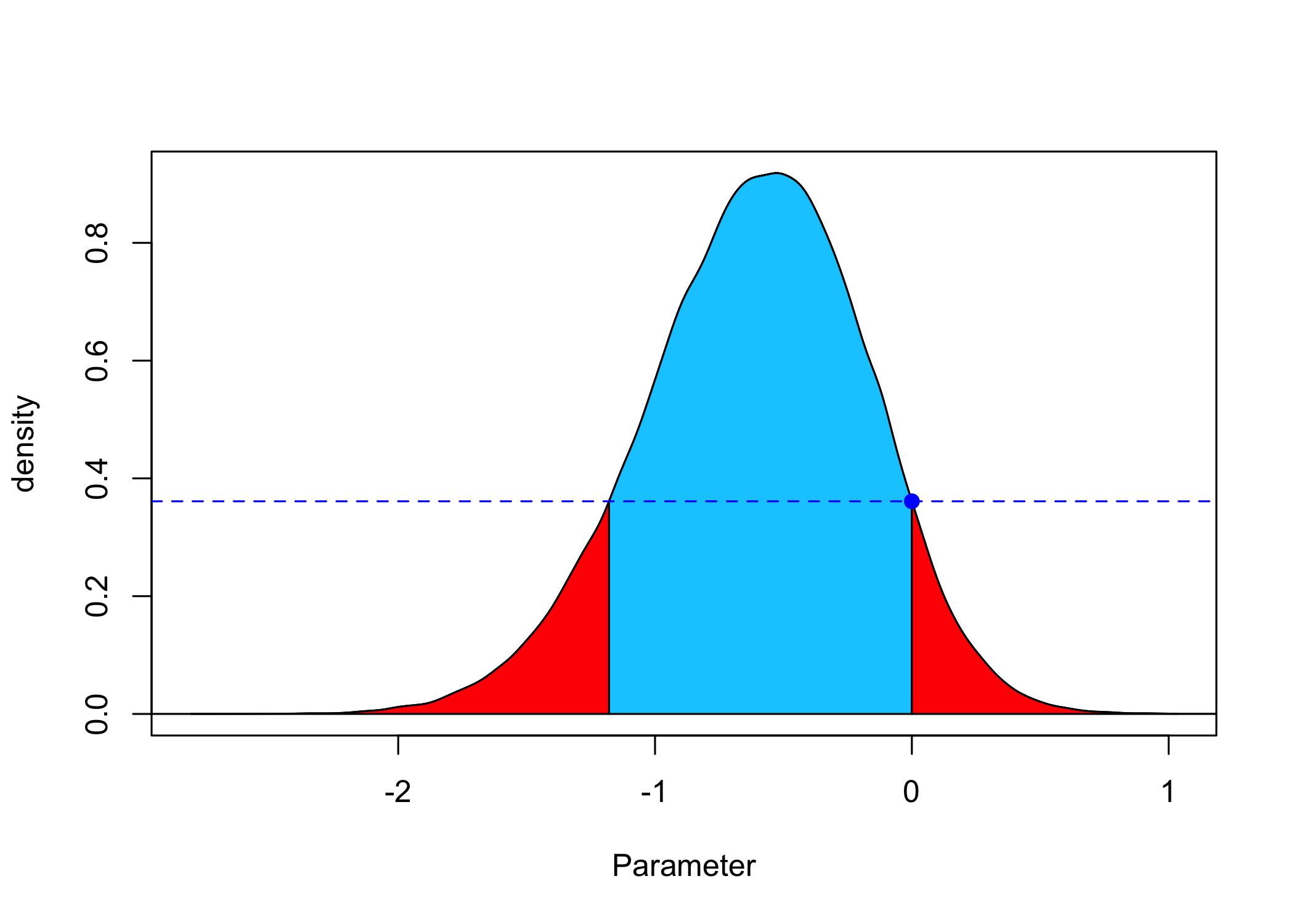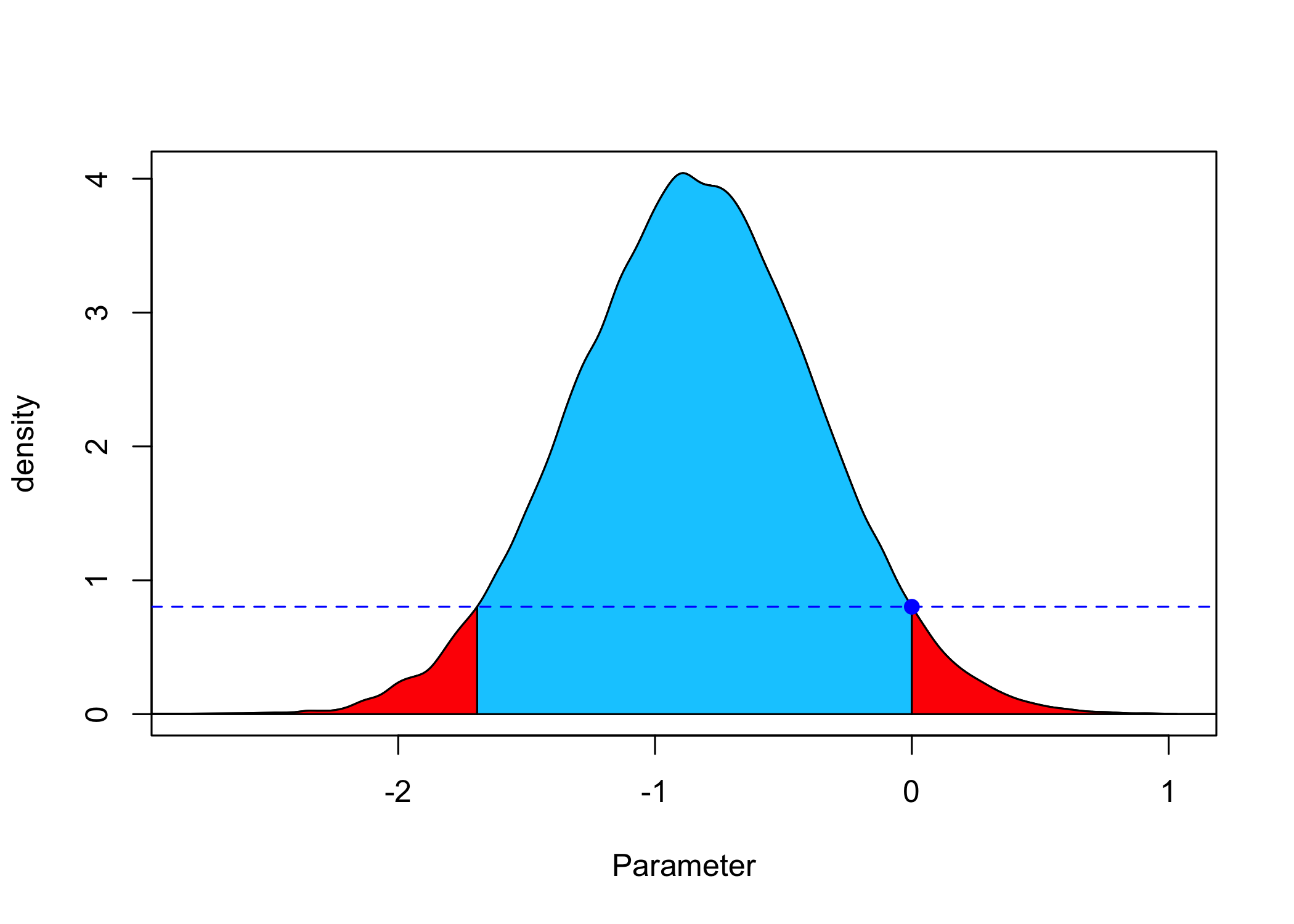# Precise Bayesian hypothesis testing with the Full Bayesian Significance Test

## Precise Bayesian hypothesis testing with the Full Bayesian Significance Test

Author: Riko Kelter

This vignette explains how to use the Full Bayesian Significance Test (FBST) for Bayesian hypothesis testing of a precise (point-null) hypothesis against its alternative via the e-value.

library(fbst)

## Theory behind the FBST and e-value

The FBST uses the e-value to quantify the evidence against a precise hypothesis. The FBST can be used with any standard parametric model, where $$\theta \in \Theta \subseteq \mathbb{R}^p$$ is a (possibly vector-valued) parameter of interest, $$p(y|\theta)$$ is the likelihood and $$p(\theta)$$ is the density of the prior distribution.

A precise hypothesis $$H_0$$ makes the statement that the parameter $$\theta$$ lies in the corresponding null set $$\Theta_{H_0}$$. For point null hypotheses like $$H_0:\theta=\theta_0$$ the null set simply is given as $$\Theta_{H_0} = \theta_0$$. The idea of the FBST is to use the $$e$$-value, which quantifies the Bayesian evidence against $$H_0$$ as a Bayesian replacement of the traditional $$p$$-value. To construct the $$e$$-value the posterior surprise function $$s(\theta)$$ is defined as follows: $s(\theta):=\frac{p(\theta|y)}{r(\theta)}$ The surprise function normalizes the posterior distribution $$p(\theta|y)$$ by a reference function $$r(\theta)$$. Possible choices include a flat reference function $$r(\theta):=1$$ or any prior distribution $$p(\theta)$$ for the parameter $$\theta$$, that is: $$r(\theta):=p(\theta)$$. In the first case, the surprise function becomes the posterior distribution $$p(\theta|y)$$, and in the second case parameter values $$\theta$$ with a surprise function $$s(\theta)\geq 1$$ indicate that they have been corroborated by the data $$y$$, while parameter values $$\theta$$ with a surprise function $$s(\theta)<1$$ indicate that they have become less probable a posteriori. $$s^{*}$$ is then defined as the supremum of the surprise function $$s(\theta)$$ over the null set $$\Theta_{H_0}$$ which belongs to the hypothesis $$H_0$$ : $s^{*}:=\sup\limits_{\theta \in \Theta_{H_0}}s(\theta)$ The tangential set $$\overline{T}(\nu)$$ to the hypothesis $$H_0$$ is defined as $\overline{T}(\nu):=\Theta \setminus T(\nu)$ where $$\Theta \setminus T(\nu)$$ is the set complement of $$\Theta$$ and $$T(\nu)$$, that is, the set which remains when removing all points in $$T(\nu)$$ from $$\Theta$$. In the above, $T(\nu):=\{\theta \in \Theta|s(\theta)\leq \nu \}$ $$T(s^{*})$$ includes all parameter values $$\theta$$ which are smaller or equal to the supremum $$s^{*}$$ of the surprise function under the null set, and $$\overline{T}(s^{*})$$ includes all parameter values $$\theta$$ which are larger than the supremum $$s^{*}$$ of the surprise function under the null set.

The last step towards the $$e$$-value is to define the cumulative surprise functions $$W(\nu)$$ and $$\overline{W}(\nu)$$: $W(\nu):=\int_{T(\nu)}p(\theta|y)d\theta$ $\overline{W}(\nu):=\int_{\overline{T}(\nu)}p(\theta|y)d\theta$ and setting $$\nu=s^{*}$$, $$W(s^{*})$$ is simply the integral of the posterior distribution $$p(\theta|y)$$ over $$T(s^{*})$$, and $$W(s^{*})$$ the integral over $$\overline{T}(s^{*})$$. Note that $$\overline{W}(\nu):=1-W(\nu)$$ due to the definition of $$T(\nu)$$ and $$\overline{T}(\nu)$$. The Bayesian $$e$$-value, which measures the evidence against the null hypothesis $$H_0$$, is then defined as $\overline{\text{ev}}(H_0):=\overline{W}(s^*)$ Thus, the e-value $$\overline{\text{ev}}(H_0)$$ is the integral $$\overline{W}(s^*)$$ of the posterior distribution over the tangential set $$\overline{T}(s^{*})$$, that is, over those values $$\theta$$ for which the surprise $$s(\theta)>s^{*}=\sup\limits_{\theta \in \Theta_{H_0}}s(\theta)$$ holds. If the probability mass of these values, which is calculated by the integral $$\overline{W}(s^*)$$, is large, this indicates substantial evidence against $$H_0$$ being a reasonable explanation of the observed data.

## Running the FBST

To run the FBST, a posterior distribution is required, which can be obtained as in any standard Bayesian workflow:

1. Specify a joint distribution for the outcome(s) and all relevant parameters. Typically, this takes the form of a marginal prior distribution for the parameters multiplied by a likelihood for the outcome(s) conditional on the parameters. This joint distribution is proportional to a posterior distribution of the parameters conditional on the observed data.
2. Run a Markov-Chain-Monte-Carlo (MCMC) algorithm to draw samples from posterior distribution.
3. Evaluate the model fit with regard to the data (e.g. via posterior predictive checks) and possibly revise the model.

Based on the sample of posterior draws, the FBST can be performed as follows:

1. Specify a null hypothesis value $$\theta_0$$ for the precise null hypothesis $$H_0:\theta=\theta_0$$.
2. Specify a reference function $$r(\theta)$$ which is used to build the surprise function $s(\theta):=\frac{p(\theta|y)}{r(\theta)}$ Typical choices include a flat reference function $$r(\theta):=1$$ which implies the surprise function becomes the posterior $$p(\theta|y)$$, or the density $$p(\theta)$$ of a prior distribution $$P_{\vartheta}$$ for the parameter $$\theta$$. In the latter case, the surprise function $$s(\theta)$$ quantifies the ratio of posterior to prior, and parameter values $$\theta$$ with $$s(\theta)\geq 1$$ can be interpreted as being corroborated by observing the data $$y$$. Parameter values $$\theta$$ with $$s(\theta)<1$$ can be interpreted as not being corroborated by observing the data $$y$$.
3. Run the FBST and compute the Bayesian evidence against $$H_0$$, the e-value $$\overline{\text{ev}}(H_0)$$.

Now, we illustrate the FBST with two examples below. The first example is a Bayesian two-sample t-test with flat reference function, and the second example the same test with a user-defined reference function.

## Examples

### Example 1: Two-sample t-test with flat reference function

We use Student’s sleep data as illustration, which is available in the data frame sleep.

sleep
#>    extra group ID
#> 1    0.7     1  1
#> 2   -1.6     1  2
#> 3   -0.2     1  3
#> 4   -1.2     1  4
#> 5   -0.1     1  5
#> 6    3.4     1  6
#> 7    3.7     1  7
#> 8    0.8     1  8
#> 9    0.0     1  9
#> 10   2.0     1 10
#> 11   1.9     2  1
#> 12   0.8     2  2
#> 13   1.1     2  3
#> 14   0.1     2  4
#> 15  -0.1     2  5
#> 16   4.4     2  6
#> 17   5.5     2  7
#> 18   1.6     2  8
#> 19   4.6     2  9
#> 20   3.4     2 10

We select both groups and perform a traditional Bayes factor two-sample t-test based on the model of Rouder et al. (2009):

library(BayesFactor)
grp1 = sleep[1:10,]$extra grp2 = sleep[11:20,]$extra
ttestBF(x=grp1,y=grp2, rscale="medium")
#> Bayes factor analysis
#> --------------
#>  Alt., r=0.707 : 1.265925 ±0%
#>
#> Against denominator:
#>   Null, mu1-mu2 = 0
#> ---
#> Bayes factor type: BFindepSample, JZS

Based on a traditional Bayes factor test, there is not much evidence for the alternative: $$BF_{10}=1.27$$. Now, we obtain posterior MCMC samples and conduct the FBST based on a flat reference function $$r(\theta)=1$$. Note that the dimension of the null set is 1 (the variances in both groups are assumed to be equal but can have any particular value in Rouder’s model), and the whole parameter space has two free parameters, namely the effect size and the variance of the groups.

posteriorDraws = as.numeric(ttestBF(x=grp1,y=grp2, rscale="medium", posterior = TRUE,
iterations = 100000)[,4])
result = fbst(posteriorDensityDraws = posteriorDraws, nullHypothesisValue = 0,
dimensionTheta = 2, dimensionNullset = 1, dim = 1)
summary(result)
#> Full Bayesian Significance Test for testing a sharp hypothesis against its alternative:
#> Reference function: Flat
#> Hypothesis H_0:Parameter= 0 against its alternative H_1
#> Bayesian e-value against H_0: 0.8307837
#> Standardized e-value: 0.05943232

The e-value $$\overline{\text{ev}}(H_0)$$ against $$H_0:\theta=0$$ is given as $$\overline{\text{ev}}(H_0)=0.83$$, so 83% of the posterior surprise function, which in this case (flat reference function) is the posterior density, attain a larger posterior density value than the posterior density value $$p(\theta_0|y)$$ at $$\theta_0=0$$. This can be visualized as follows:

plot(result)The blue point shows the resulting surprise function value at $$\theta_0=0$$. As the flat reference function $$r(\theta)=1$$ is used, $$s(\theta)=p(\theta|y)$$, so the blue point is the posterior density value $$p(0|y)$$. The blue area shows the associated probability mass with the parameter values $$\theta$$ which attain a larger surprise than the surprise at $$\theta_0$$, that is, the tangential set to $$H_0$$. Thus, these values $$\theta$$ fulfill $$s(\theta)>s(0)$$ which is equivalent to $$p(\theta|y)>p(0|y)$$ in this case. The red area shows the probability mass associated with values $$\theta$$ that do not fulfill this condition. Based on the result there is evidence for the alternative, but there are about $$16\%$$ of the posterior which prevent a conclusive statement or clear rejection of $$H_0:\theta=0$$.

### Example 2: Two-sample t-test with user-specified reference function

In the above, a flat reference function $$r(\theta)=1$$ was used in the surprise function $$s(\theta)$$. However, the prior distribution on the parameter $$\theta$$ was a medium Cauchy prior $$C(0,\sqrt{2}/2)$$. As the prior beliefs are reflected via this prior, it is reasonable to choose the Cauchy prior as the reference function instead. Below, it is shown how to do this:

result2 = fbst(posteriorDensityDraws = posteriorDraws, nullHypothesisValue = 0,
dimensionTheta = 2, dimensionNullset = 1, FUN = dcauchy,
par=list(location = 0, scale = sqrt(2)/2))
summary(result2)
#> Full Bayesian Significance Test for testing a sharp hypothesis against its alternative:
#> Reference function: User-defined
#> Hypothesis H_0:Parameter= 0 against its alternative H_1
#> Bayesian e-value against H_0: 0.9215442
#> Standardized e-value: 0.0240581
plot(result2)Now, the result changes. The e-value $$\overline{\text{ev}}(H_0)=0.92$$ now shows stronger evidence against $$H_0$$. While the two plots look very similar, note the different scaling at the y-axis.

## A note on standardized e-values

The output above also reports the standardized e-values $$\text{sev}(H_0)$$ as part of the analysis via the FBST. The standardized e-value is a modified version of the plain e-value $$\overline{\text{ev}}(H_0)$$.

The standardized $$e$$-value is defined as: $\overline{\text{sev}}(H_0)=F_{k-h}(F^{-1}_{k}(\overline{\text{ev}}))$ Here, $$F^{-1}_{k}$$ is the quantile function of the cumulative distribution function of the $$\chi_{k}^2$$ distribution with $$k$$ degrees of freedom. $$\overline{\text{sev}}(H_0)$$ can be interpreted as the probability of obtaining less evidence than $$\overline{\text{ev}}(H_0)$$ against the null hypothesis $$H_0$$. Defining $\text{sev}(H_0)=1-\overline{\text{sev}}(H_0)$ $$\text{sev}(H_0)$$ can then be interpreted as the probability of obtaining $$\overline{\text{ev}}(H_0)$$ or more evidence against $$H_0$$, which is closely related to the interpretation of a frequentist $$p$$-value. If $$\text{sev}(H_0)$$ is small, this implies that the probability of obtaining even more evidence than $$\overline{\text{ev}}(H_0)$$ against the null hypothesis $$H_0$$ is small. As a consequence, one can reject $$H_0$$. However, the $$p$$-value operates in the sample space while the standardized $$e$$-value operates in the parameter space. The standardized $$e$$-value can be used as a Bayesian replacement of the frequentist $$p$$-value, while being very similar in interpretation.

result$sev_H_0 #> sev_H_0 #> 0.05943232 result2$sev_H_0
#>   sev_H_0
#> 0.0240581

In the first example, we would thus not reject $$H_0:\theta=0$$ based on a threshold of $$\text{sev}(H_0)<0.05$$, while in the second example above we would. Note the identical trend in the Bayesian evidence against $$H_0:\theta=0$$ based on the non-standardized e-values $$\overline{\text{ev}}(H_0)$$. For details on the theoretical properties of the standardized e-value, see Kelter (2020) and Pereira & Stern (2020).

## Conclusion

In this vignette it was demonstrated how to perform the FBST for testing a precise hypothesis in the Bayesian paradigm. It was shown that the FBST can be applied to a wide range of statistical models as long as the posterior is obtainable via standard MCMC algorithms. Thus, the FBST presents a flexible approach to Bayesian hypothesis testing while being fully coherent with the likelihood principle and the associated benefits (e.g. the irrelevance of stopping rules, the irrelevance of censoring mechanisms).

When an interval hypothesis is more reasonable, the Full Bayesian Evidence Test (FBET) presents a generalization of the FBST and the underlying Pereira-Stern theory and is also available in the package.